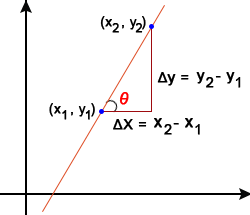# Slope Calculator

Slope Calculator allows you to find the slope of line between given two points in the cartesian coordinate system.

##### More Useful Tools
Square Root Calculator Inverse Matrix Calculator

Slope Calculator is an online geometry tool. It takes two-dimensional coordinate points (x1, y1) and (x2, y2) as an input. As a result, it gives the Slope of Line passing through those points.### What is Slope?

It's the amount of slant which the line has. Also, its value can be zero, positive, negative, or undefined. Most importantly, we need two coordinate point values to find the slope.

#### Slope Formula

You can easily find the slope of a line using the following formula.

m =
 y2 - y1 x2 - x1
=
 ΔY ΔX
=  tan(θ)

Where,

m = Slope

(x1, y1) and (x2, y2) = Coordinate Points

tan(θ) = Angle of Incline### How to Find Slope?

Let's take an example to understand the calculation process.

#### Example:

Find the slope of line that passes throght the Coordinate Points (x1, y1) = (5, 10) and (x2, y2) = (8, 18).

Firstly, let's define all the values.

Here,
x1 = 5
y1 = 10
x2 = 8
y2 = 18

Now place above all values in slope formula.

m =
 y2 - y1 x2 - x1
m =
 18 - 10 8 - 5
=
 8 3

= 2.6667

So, we get slope(m) = 2.6667.

It's very simple to solve the equation for small numbers. But it becomes complex when we are dealing with big numbers. So, in this situation, you can use our slope calculator and make complex calculations easy and fast.

#### Distance Formula

Distance (d) = √(ΔX2 + ΔY2)

OR

Distance (d) = √(x2 - x1)2 + (y2 - y1)2

### How to use Slope Calculator?

It's very simple to use our calculator. Just follow the steps below.

1. Firstly, it's an online tool. So, you need an Internet connection and web browser to get started.
2. Open the calculator in any web browser like Chrome, Firefox, Microsoft Edge, etc.
3. You can see four empty boxes at the top of the page.
4. Those are the Coordinate points and it asks you to enter the values of X1, Y1, X2, and Y2. So, enter the correct values in appropriate field.
5. Lastly, press the "Calculate" button.
6. As a result, you will get the final result of Slope (m), Angle (θ), Distance (d), ΔX, and ΔY just below the tool.